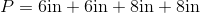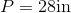# ISEE Upper Level Math : How to find the perimeter of a parallelogram

## Example Questions

### Example Question #5 : Parallelograms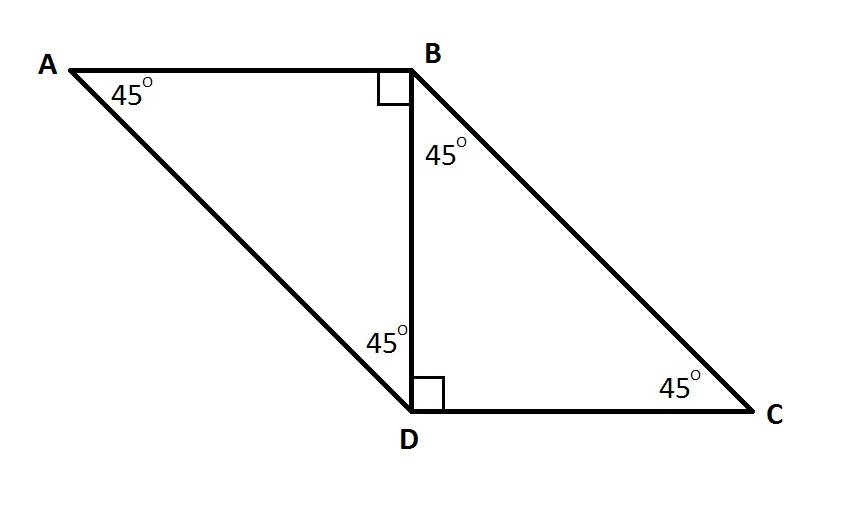The area of the Parallelogramis. Give its perimeter in terms of.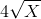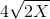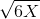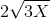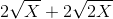Explanation:

The height of the parallelogram is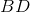, and the base is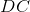. By the 45-45-90 Theorem,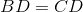. Since the product of the height and the base of a parallelogram is its area,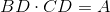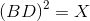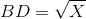Also by the 45-45-90 Theorem,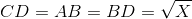, and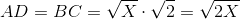The perimeter of the parallelogram is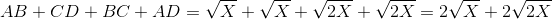### Example Question #6 : ParallelogramsCalculate the perimeter of the above parallelogram if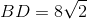.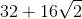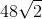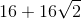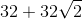Explanation:

By the 45-45-90 Theorem,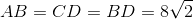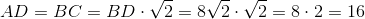The perimeter of the parallelogram is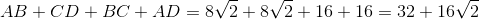### Example Question #7 : Parallelograms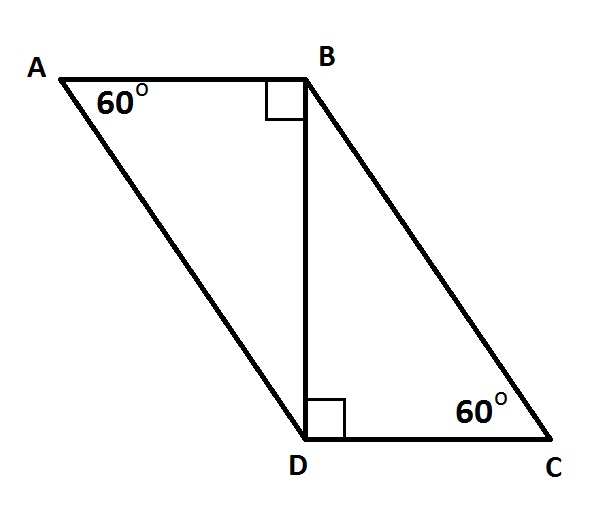Calculate the perimeter of the above parallelogram if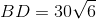.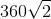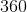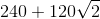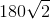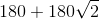Explanation:

By the 30-60-90 Theorem: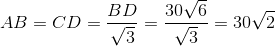, and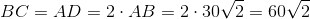The perimeter of the parallelogram is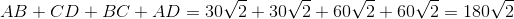### Example Question #8 : Parallelograms

Find the perimeter of a parallelogram with a base of 6in and a side of length 8in.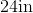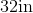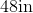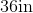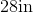Explanation:

A parallelogram has 4 sides.  A base (where the opposite side is equal) and a side (where the opposite side is equal).  So, we will use the following formula: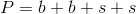where b is the base and s is the side of the parallelogram.

We know the base has a length of 6in.  We also know the side has a length of 8in.

Knowing this, we can substitute into the formula.  We get